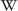The hexadecimal number system is a denominational number system on the base of 16.

## General

The hexadecimal number system is a denominational number system on the base of 16. To represent the values zero (0) through fifteen (15), the ten, common, decimal digits (0 thru 9) are supplemented with the alphabetic letters A thru F to represent the equivalent decimal values 10 thru 15. Thus hexadecimal digits range from zero (0) through 'F'.

Every four binary-coded digits can be represented as a single hexadecimal digit. A single hexadecimal digit (0-F) represents a half-byte or nibble and each pair of hexadecimal digits represents a full byte. Hexadecimal notation is often used in machine programming languages e.g. Assembler, to represent memory addresses or memory contents.

Hexadecimal is often distinguished from decimal numbers with a prefix such as "0x" or "&h", or a postfix (ending) such as "h". The C64 typically uses the Dollar symbol ("\$") as the hexadecimal prefix (e.g. \$8000). In mathematical representations, the hexadecimal number receives a subscript in the form of a lowered (16). For example: dec. 123 = bin. 011110112 = \$7B = &h7B = 7Bh = 0x7B = 7B16.

The advantage of the hexadecimal system in working with computers is that from the view of the computer "even" binary numbers such as 1000000002 look awkward when represented in the decimal system (256(10) in this case). The same number in hexadecimal form appears relativly readable as 100(16).

(as Nibble)

Decimal no.
0 0000 0
1 0001 1
2 0010 2
3 0011 3
4 0100 4
5 0101 5
6 0110 6
7 0111 7
8 1000 8
9 1001 9
A 1010 10
B 1011 11
C 1100 12
D 1101 13
E 1110 14
F 1111 15

## Conversion

For programmers are interesting conversion methods, e.g. how could be a hexadecimal number be converted in a decimal number or which decimal number results from the existing hexadecimal number.

For example we will use the binary number 9F.
In advance the F must be implemented in the formula as 15:

`PRINT "DECIMAL NO.: "9*16↑1 + 15*16↑0"`
And the monitor output says: DECIMAL NO: 159

The conversion from decimal to hexadecimal is a little bit more difficult. A simple method is the division method. By this method the decimal number will be so long divided through the base 16 until the result is 0, at which the rest will be noticed which reverse read in the hexadecimal number results. Additional it must be considered that the rest 10 to 15 must be conversed in the hex digits A to F.

```159 : 16 = 9 Rest  ..15
009 : 16 = 0 Rest  .9
-----------------------
```

As BASIC programs:

Easy variant
```10 PRINT "=== DECIMAL TO HEXADECIMAL ==="
20 INPUT "DECIMAL NO.:";A\$
30 A = ABS( VAL(A\$) )
40 B = INT(A/16)
41 A = A - (B*16)
50 IF A=10 THEN C\$ =C\$ +"A"
51 IF A=11 THEN C\$ =C\$ +"B"
52 IF A=12 THEN C\$ =C\$ +"C"
53 IF A=13 THEN C\$ =C\$ +"D"
54 IF A=14 THEN C\$ =C\$ +"E"
55 IF A=15 THEN C\$ =C\$ +"F"
56 IF A<10 THEN C\$ =C\$ +MID\$( STR\$(A),2,1 )
57 A = B : IF B<>0 THEN 40
60 FOR X = LEN(C\$) TO 1 STEP -1
61 B\$ = B\$ + MID\$(C\$,X,1)
62 NEXT X : PRINT "HEXADECIMAL:" B\$
```

Comment: The program works in 2 parts. In the rows 40 to 57 the division is made and in rows 60 to 61 the reversion (from the wrong cached result F9 will be made the right result 9F).

Professional variant
```10  PRINT "=== DEC TO HEX  PRO. ==="
20  INPUT "DECIMAL NO.:";A\$
30  A = ABS( VAL(A\$) )
40  B = INT(A/16)
50  A = A - (B*16)
60  IF A>9 THEN C\$ =C\$ +CHR\$(55+A)
70  IF A<10 THEN C\$ =C\$ +MID\$( STR\$(A),2,1 )
80  A = B : IF B<>0 THEN 40
90  FOR X = LEN(C\$) TO 1 STEP -1
100 B\$ = B\$ + MID\$(C\$,X,1)
110 NEXT X : PRINT "HEXADECIMAL:" B\$
```

Comment: This version is a modify variant of the "easy program version". On line 60, we use the knowledge that character code 65="A" for our benefit.

Real Programmer's Variant
```10 PRINT "=== DEC TO HEX REAL PRO ==="
20 INPUT "DECIMAL NO.:";A\$
30 V% = ABS( VAL(A\$) )
40 GOSUB 100 : PRINT "HEXADECIMAL: " R\$
50 END
100 R\$ = "" : HX\$ = "0123456789ABCDEF"
110 R\$ = MID\$(HX\$, 1+(V% AND 15), 1) + R\$
120 V% = V%/16 : IF V%=0 THEN RETURN
130 GOTO 110
```

Comment: This version makes use of Boolean algebra: It extracts the lowest 4 bits (which can be 0 to 15) from V and uses it as index into the string HX\$, then divides V by 16, effectively shifting all bits 4 places to the right, until nothing is left to convert (V=0).

Long binary numbers are simply splitted from right to left in 4 Bit Units. From these you can easily convert with the above table the hexadecimal numbers:

```1011.0010.1100.1001 entspricht B2C9
```

```F3A6 equates to 1111.0011.1010.0110Wikipedia: Hexadecimal